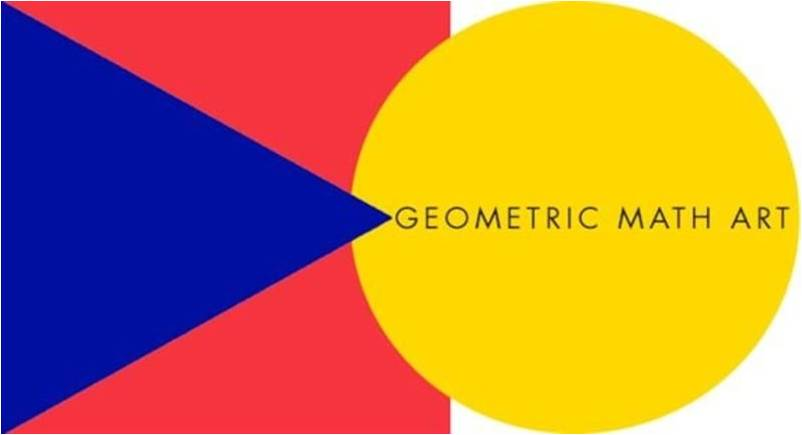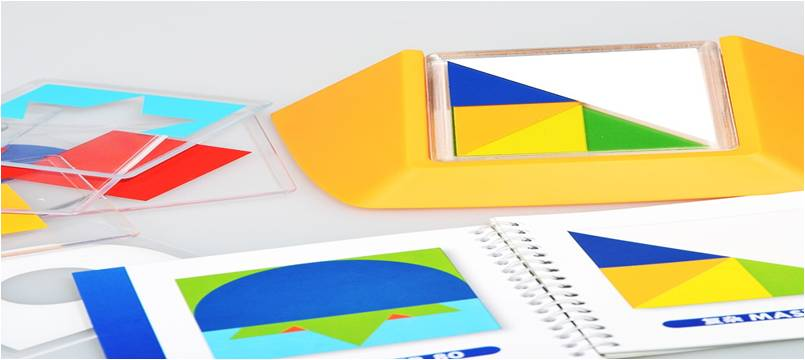# Geometry Curriculum

#### LOWER ZONE GEOMETRY CURRICULUMAs for lower zone students, the study of geometry continues to spiral and go into more depth. They explore with the measurement of area and volume, introduction to Pythagorean and Euclidian geometry. Other topics in geometry includes:

• Geometric plane figures
• Circles
• Squares and rectangles
• Triangle
• Polygons
• Curved figures
• Study of geometric solids
• Basic concepts of lines and angles
• Measurement: perimeter

#### UPPER ZONE GEOMETRY CURRICULUM

Students in the upper zone, they are introduced to three great concepts of Geometry known as congruence, similarity and equivalence. These are referred as “Golden Elements” of geometry. At this level, they explore with other topics such as:

• Geometric shapes: characteristics, measurements, and drawing
• Concepts such as Points, lines, angles, surface and solids
• Properties – Triangles and quadrilaterals, Polygons and circles
• Congruence, similarity, equality, equivalence, symmetry
• Calculation: perimeter, area, volume
• Theorem of Pythagoras
• Measurement: length, weight, capacity, metric and customary